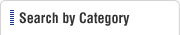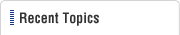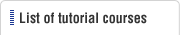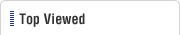HOME  > Plastic Molding Tutorial  > #139 Strength Calculation of the Thickness of the Side Wall of a Cavity (In the case of a rectangular cavity with bottom surface parting)
Plastic Molding Tutorial

#139 Strength Calculation of the Thickness of the Side Wall of a Cavity (In the case of a rectangular cavity with bottom surface parting)

Category : Cavity
February 1, 2013

While in the last lesson we have explained the method of determining the external dimensions of a cavity (fixed side inserts), from this lesson onwards we explain the actual calculation of the strength of the thickness of the side wall of a cavity.

This time we shall take the example of calculating the side wall thickness of a rectangular cavity whose bottom surface is parted.

The shape of the cavity in this example is shown in Fig.1.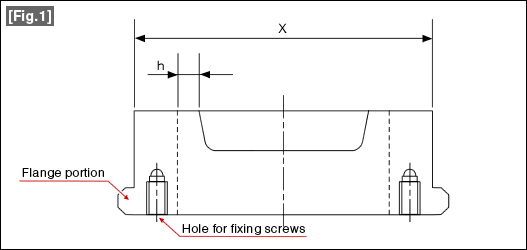The thickness h of the side wall in Fig. 1 can be obtained using the following equation.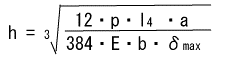Here,
h:Thickness of the cavity wall (mm),
p:Cavity internal pressure (kgf／cm2),
l:Inside length of the cavity (mm),
a:Height of the side wall receiving the cavity internal pressure p (mm),
b:Height of the cavity (mm),
E:Modulus of elasticity (Young's modulus) (kgf/cm2)(kgf／cm2),
σmax:Maximum permissible strain.(mm)

Calculate the values of each of the above variables according to the units within parentheses ().

The internal pressure p of the cavity takes on values in the range of 200 to 600 kgf/cm2.樹The value of p varies depending on the plastic and the molding conditions. For example, the value will be about 400 kgf/cm2in the case of ABS plastic, and will be about 600 kgf/cm2the case of PC plastic.

l is the length of the periphery of the cavity,
a is the depth of the periphery of the cavity, and
b is the height of the cavity,
E is the modulus of elasticity of the steel material of the cavity.
See the data in some back numbers for the value.

σmax is the maximum permissible strain of the side wall. A value of 0.01 to 0.02 mm is appropriate.

The data substituted in the equation for calculation should be determined considering the molding conditions or the type of steel, etc.

In the next lesson, we will carry out actual calculations for an example.